• 二阶rc低通滤波器
万次阅读 多人点赞
2019-07-19 10:50:09

# RC电路原理推导

因为最近有做一些RC滤波电路的东西，这部分内容都是在大学本科的时候学习的，很多东西也记得不是很清晰了，手头也没有资料翻阅，在网上看的资料都是五花八门各不一样，很多都出现错误，并且对于二阶RC电路的介绍很少，所以我做了一些整理。

## 一阶RC低通滤波电路

一阶RC低通滤波电路如下图所示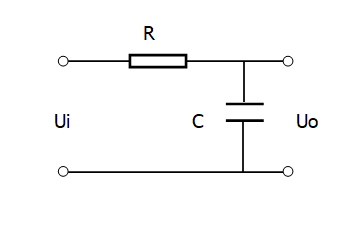对应系统的传递函数的表达式如下 U o U i = 1 R C S + 1 \frac{\mathrm{U}_{\mathrm{o}}}{\mathrm{U}_{\mathrm{i}}}=\frac{1}{R C S+1}
对其进行离散化处理 S = 1 − z − 1 T S=\frac{1-z^{-1}}{T}
可得 R C ⋅ 1 − z − 1 T R C 1 − z − 1 T + 1 = R C ( 1 − z − 1 ) R C ( 1 − z − 1 ) + T = Y n X n \frac{R C \cdot \frac{1-z^{-1}}{T}}{R C \frac{1-z^{-1}}{T}+1}=\frac{R C\left(1-z^{-1}\right)}{R C\left(1-z^{-1}\right)+T}=\frac{Y_{n}}{X_{n}}
整理可得 Y n = T T + R C X n + R C T + R C Y n − 1 Y_{n}=\frac{T}{T+R C} X_{n}+\frac{RC}{T+R C} Y_{n-1}
以上就是我们常见的一阶滤波的形式，转换成我们常见的表达式就是 Y n = a X n + ( 1 − a ) Y n − 1 Y_{n}=a X_{n}+(1-a) Y_{n-1}

## 二阶RC低通滤波电路

好了讲完一阶来讲二阶，二阶相对于一阶计算的时候要复杂一点，思路还是一样的，二阶RC低通滤波的电路如下所示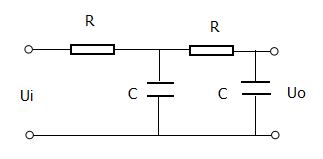二阶RC低通滤波器的传递函数表达式为 U 0 U i = 1 S C / / ( R + 1 S C ) R + 1 S C / ( R + 1 S C ) ⋅ 1 S C R + 1 S C \frac{U_{0}}{U_{i}}=\frac{\frac{1}{S C} / /\left(R+\frac{1}{SC}\right)}{R+\frac{1}{SC} /\left(R+\frac{1}{SC}\right)} \cdot \frac{\frac{1}{SC}}{R+\frac{1}{SC}}
简化后可得 U 0 U i = R C S + 1 R 2 C 2 S 2 + 3 R C S + 1 ⋅ 1 R C S + 1 = 1 R 2 C 2 S 2 + 3 R C S + 1 \frac{U_{0}}{U_{i}}=\frac{RCS+1}{R^{2} C^{2} S^{2}+3 R C S+1} \cdot \frac{1}{R C S+1}=\frac{1}{R^{2} C^{2} S^{2}+3 R C S+1}
离散化 S = 1 − z − 1 T S=\frac{1-z^{-1}}{T} 可得 T 2 R 2 C 2 ( 1 − z − 1 ) 2 + 3 R C ( 1 − z − 1 ) + T 2 = Y n X n \frac{T_{}^{2}}{R^{2} C^{2}\left(1-z^{-1}\right)^{2}+3 R C\left(1-z^{-1}\right)+T^{2}}=\frac{Y_{n}}{X_{n}} 整理后可得 Y n = T 2 R 2 C 2 + T 2 + 3 R C X n + 2 R 2 C 2 + 3 R C R 2 C 2 + T 2 + 3 R C Y n − 1 + − R 2 C 2 R 2 C 2 + T 2 + 3 R C Y n − 2 Y_{n}=\frac{T^{2}}{R^{2} C^{2}+T^{2}+3 RC}X_{n}+\frac{2 R^{2} C^{2}+3 R C}{R^{2} C^{2}+T^{2}+3 R C} Y_{n-1}+\frac{- R^{2} C^{2}}{R^{2} C^{2}+T^{2}+3 R C} Y_{n-2} 可以看出二阶RC与除了与当前时刻的输入有关系还和上两个时刻的输出有关。一阶二阶RC 低通滤波
更多相关内容
• 需求分析 我们这里使用二阶RC低通滤波来达到检流电阻的PWM波等效DC直流电平的功能； 电路示意图如下,这里的PWM不是MCU直接输出的，而是经过推挽放大电路处理过的信号 R11/R12 、C3/C4组成二阶RC滤波；这里R11=R12、...

1.原理及用途

(1)原理直接引用*原子STM32讲解PWM转DAC的篇章*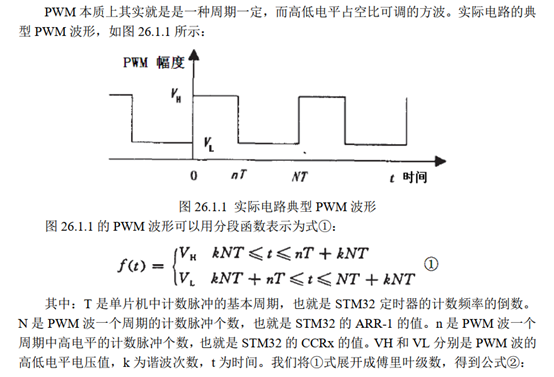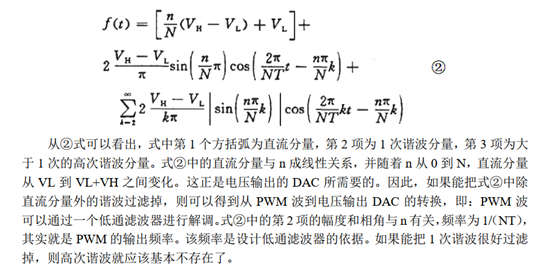(2)当MCU中DAC功能不够用时，而还要输出可控的DC电平，这时可以使用这种模式来达到目的；另外一些由PWM控制的负载器件，比如比例阀、电机，要测试这些负载流过的等效电流，也可以使用这种方法

2.需求分析

我们这里使用二阶RC低通滤波来达到检流电阻的PWM波等效DC直流电平的功能；

电路示意图如下,这里的PWM不是MCU直接输出的，而是经过推挽放大电路处理过的信号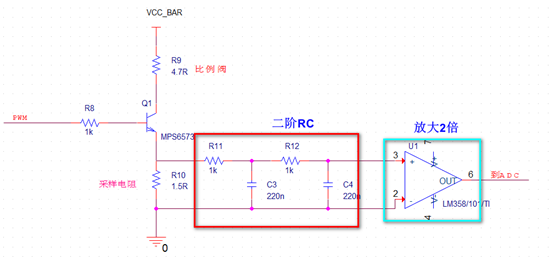R11/R12 、C3/C4组成二阶RC滤波；这里R11=R12、C3=C4

3.数学计算

假如我们要求经过滤波后结果达到检测电流±1mA，则1个位的精度达到1.5/2048=0.0007324V，采样电阻电平范围0-1.5V

假设被滤波的信号电压范围0-1.5V,那么一次谐波的最大值1.5*2/π=0.95496,那么电路要至少提供-20log(0.95496/0.0007324V)=-63dB衰减

这里使用的PWM是F=20KHz

如果是一阶RC滤波，那截止频率Fs的计算由-10*log[1+(F/Fs)^2]=-63dB，得Fs=14Hz

如果是二阶RC滤波，那截止频率Fs的计算由-20*log[1+(F/Fs)^2]=-63dB，得Fs=532Hz

参数RC选择：

这里使用二阶低通滤波器，Fs=1/2πRC，得R=1KΩ,C=300nF（这里是由设计者选取），(R11=R12=R,C3=C4=C),这个RC乘积是个定值，至于R  C选择组合，可根据实际应用来确定，但是有一个思路：R或C不能太小，比如R取很大时，C必然取小，这时由于电路的寄生效应，导致最后的等效电容增大比例很多，会影响滤波效果，这样就不行了；反过来选取R一样

一阶二阶对比，除了电路上不同；计算的截止频率差别也是很大

二阶衰减比一阶严重一点、但是二阶延迟较小，而且一阶纹波大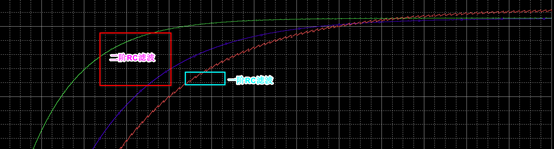4.电路仿真及波形数据-二阶滤波器

因为实际负载工作电流范围：0-1A，故而电流电阻上的电压范围0-1.5V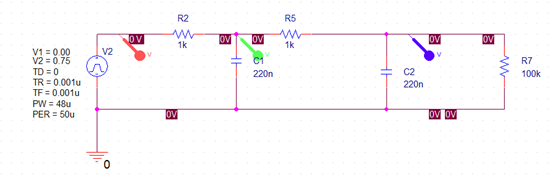V1是输出最小电平

V2是输出最大电平，实际在V1-V2之间选择来试验

TD是PWM波延迟时间

TR是边沿上升时间

TF是边沿下降时间

PW占空比

PER周期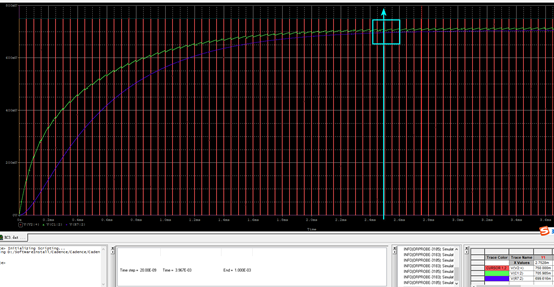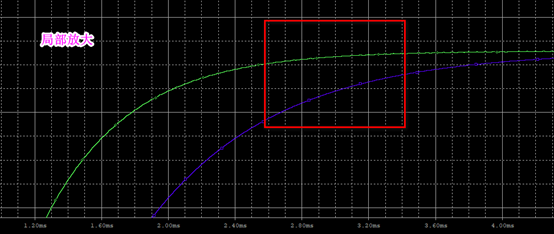由图可知绿色波形在t=3-4ms时达到稳定，最大有3-4ms的延迟（按照100%计算）

实际的RC参数确定，要根据输出的延迟时间、纹波大小来综合考量；当然这个只是仿真，到实际硬件电路中，你会发现还要有更改

仿真波形中，你会发现滤波后的波形会有衰减，这个要进行补偿

5.硬件实验波形及数据

最后选定参数R=1kΩ、C=220nF 实际硬件测得的波形如下：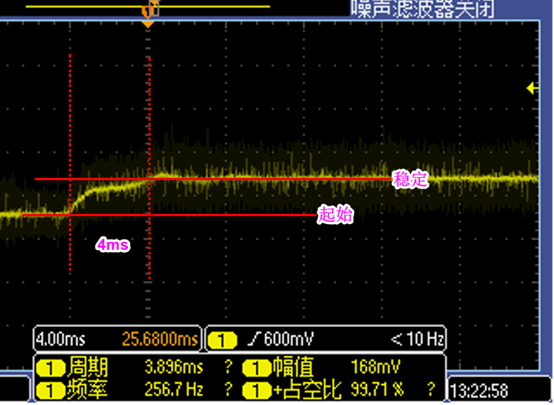仿真结果如下：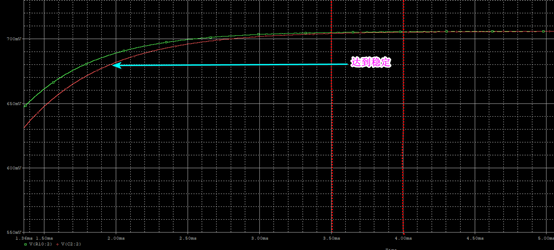对比波形，仿真和实际硬件测试基本上一致

展开全文• 1 引 言　本文引用地址：http://www.eepw.com.cn/article/270617.htm　　Multisim是加拿大Interactive Image Technologies公司近年推出的电子线路仿真软件EWB(Electronics Workbench，虚拟电子工作平台)的升级版。...
• 设计二阶低通滤波器，电源电压± 5V，要求完成下面的技术指标： a. 滤波器的截止频率fH ≤100HZ； b. 通带电压增益Au ≥ 1　；
• 利用Multisim可以实现从原理图到PCB布线工具包(如Electronics Workbench的Ultiboard)的无缝隙数据传输，且界面直观，操作方便。
• 二阶低通滤波器的设计一、系统设计方案选择1、总方案设计方案框图2、子框图的作用RC网络的作用在电路中RC网络起着滤波的作用，滤掉不需要的信号，这样在对波形的选取上起着至关重要的作用，通常主要由电阻和电容组成...

二阶低通滤波器的设计

一、系统设计方案选择

1、总方案设计

方案框图2、子框图的作用

RC网络的作用

在电路中RC网络起着滤波的作用，滤掉不需要的信号，这样在对波形的选取上起着至关重要的作用，通常主要由电阻和电容组成。

放大器的作用

电路中运用了同相输入运放，其闭环增益RVF=1+R4/R3同相放大器具有输入阻抗非常高，输出阻抗很低的特点，广泛用于前置放大级。

反馈网络的作用

将输出信号的一部分或全部通过牧电路印象输入端，称为反馈，其中电路称为反馈网络，反馈网络分为正、负反馈。3、方案选择

1)滤波器的选择

一阶滤波器电路最简单，但带外传输系数衰减慢，一般在对带外衰减性要求不高的场合下选用。无限增益多环反馈型滤波器的特性对参数变化比较敏感，在这点上它不如压控电压源型二阶滤波器。

2)级数的选择

滤波器的级数主要根据对带外衰减特殊性的要求来确定。每一阶低通或高通电路可获得-6dB每倍频程(-20dB每十倍频程)的衰减，每二阶低通或高通电路可获得-12dB每倍频程(-40dB每十倍频程)的衰减。

3)元器件的选择

一般设计滤波器时都要给定截止频率fc(ωc)带内增益Av，以及品质因数Q(二阶低通或高通一般为0.707)。在设计时经常出现待确定其值的元件数目多于限制元件取值的参数之数目，因此有许多个元件均可满足给定的要求，这就需要设计者自行选定某些元件值。一般从选定电容器入手，因为电容标称值的分档较少，电容难配，而电阻易配，可根据工作频率范围按照表1.1.3初选电容值。

二、系统组成及工作原理

1、有源二阶压控滤波器

基础电路如图1所示图1二阶有源低通滤波基础电路

它由两节RC滤波电路和同相比例放大电路组成，在集成运放输出到集成运放同相输入之间引入一个负反馈，在不同的频段，反馈的极性不相同，当信号频率f＞＞f0时(f0为截止频率)，电路的每级RC电路的相移趋于-90º，两级RC电路的移相到-180º，电路的输出电压与输入电压的相位相反，故此时通过电容c引到集成运放同相端的反馈是负反馈，反馈信号将起着削弱输入信号的作用，使电压放大倍数减小，所以该反馈将使二阶有源低通滤波器的幅频特性高频端迅速衰减，只允许低频端信号通过。其特点是输入阻抗高，输出阻抗低。2、无限增益多路反馈有源滤波器

基本形式图在二阶压控电压源低通滤波电路中，由于输入信号加到集成运放的同相输入端，同时电容C1在电路参数不合适时会产生自激震荡。为了避免这一点，Aup取值应小于3.可以考虑将输入信号加到集成运放的反相输入端，采取和二阶压控电压源低通滤波电路相同的方式，引入多路反馈，构成反相输入的二阶低通滤波电路，这样既能提高滤波电路的性能，也能提高在f=f0附近的频率特性幅度。由于所示电路中的运放可看成理想运放，即可认为其增益无穷大，所以该电路叫做无限增益多路反馈低通滤波电路。三、单元电路设计、参数计算、器件选择

1、二阶压控低通滤波器设计及参数计算所以根据上述推导公式可得：电路设计时应该使得，根据市场能买到的器件，则可以取R4+R3+10k，然后由中心频率计算公试可以取C1=C2=0.1uF，可以得出电阻R1=596.58k，R4=1.06255k.可以用2k的电位器代替，基本达到设计要求了。

仿真电路图如下所示：

2、无限增益多路反馈有源滤波器的设计及参数计算根据上述推导公式可得：电路设计时应该使得C1=C2，根据市场能买到的器件，则可以取C1=C3=0.1uF，然后由中心频率计算公式，电压增益公式以及品质因素的公式计算参数，依据以上三个公式，取fO=2KHz，Q=0.707，Aup=2.令R1=R2可得：R1=R2=0.22519K，3R=0.45038K，而用R1，R2用2K的电位器调节，

使得其等于0.22519K即可基本达到设计要求。其仿真电路图如下四、电路组装及调试

1、压控电压源二阶低通滤波电路当输入的信号频率小于截止频率2000hz，其电路的增益为2.即其波形的峰峰值是两倍2、无限增益多路负反馈二阶低通滤波器

其仿真电路图如上：

当输入的频率是1000HZ，2000HZ，30000JHZ的交流电源是输出信号的波形图分别如下：

输出与输入的倍数关系分别是2倍，1.4倍然后是截至了，趋于0.滤波器的滤波效果已经达到，截至频率是2000HZ。小于2000HZ时，输出波放大2倍。展开全文• 一个二阶低通滤波器包含两个RC支路，如图所示为二阶低通滤波器的一般电路。此一般电路对于二阶高通滤波器也同样适用。 图6－2－3所示的滤波器是同相放大器。在图6－2－3中，零频增益为 在节点B可得 　将式（6－...
• 本设计有源低通二阶滤波器(又称Sallen-Key 低通滤波器)使用公式法计算得到各参数,本设计从运算放大器的输出到同相端输入间引入一个负反馈构建而成。本文详细叙述了如何用计算法设计有源低通二阶滤波器的方法，并用...
• iir低通数字滤波器c语言设计 通过Easy-x插件画图 程序有一个错误,生成原始波形的储存位置应该改成e盘
• ## RC低通滤波器截止频率公式推导

万次阅读 多人点赞 2022-01-11 14:55:56
​=22 ​​ 化简可得， ω R C = 1 = 2 π f c R C \omega RC=1=2\pi f_cRC ωRC=1=2πfc​RC 求得截止频率 f c f_c fc​， f c = 1 2 π R C f_c=\frac{1}{2\pi RC} fc​=2πRC1​ 二阶RC低通滤波器 二阶RC滤波器如...

## 一阶RC低通滤波器一阶RC滤波器如图所示，电阻 R R 串联电容 C C ，输入电压记为 U i U_i ，输出电压记为 U o U_o ，电容容抗记为 X c = 1 j ω c X_c=\frac{1}{j\omega c}

根据串联分压，列出传递函数，
H ( j ω ) = U o U i = X c R + X c = 1 j ω c R + 1 j ω c = 1 1 + j ω c H(j \omega)=\frac{U_o}{U_i}=\frac{X_c}{R+X_c}=\frac{\frac{1}{j\omega c}}{R+\frac{1}{j\omega c}}=\frac{1}{1+j\omega c}
复数为分母，实数为分子，不方便我们后续计算复向量的模，这里做一下简单的变换，给出变换的过程。
假设有复数 Z = a + b j Z=a+bj ，则倒数为 1 Z = 1 a + b j \frac{1}{Z}=\frac{1}{a+bj} ，分子分母同乘以 a − b j a-bj ，即有，
1 Z = a − b j ( a + b j ) ( a − b j ) = a − b j a 2 + b 2 \frac{1}{Z}=\frac{a-bj}{(a+bj)(a-bj)}=\frac{a-bj}{a^2+b^2}
带入可得，
H ( j ω ) = U o U i = 1 − j ω R C 1 + ( ω R C ) 2 H(j \omega)=\frac{U_o}{U_i}=\frac{1-j\omega RC}{1+(\omega RC)^2}

计算该复数的模，则有，
∣ H ( j ω ) ∣ = ( 1 1 + ( ω R C ) 2 ) 2 + ( ω R C 1 + ( ω R C ) 2 ) 2 = 1 1 + ( ω R C ) 2 |H(j \omega)|=\sqrt{(\frac{1}{1+(\omega RC)^2})^2+(\frac{\omega RC}{1+(\omega RC)^2})^2}=\sqrt{\frac{1}{1+(\omega RC)^2}}
复数的模代表了电压增益，当电压增益下降到 2 2 \frac{\sqrt2}{2} 倍时，此时的频率即为截止频率，记为 f c f_c ，那么就有，
∣ H ( j ω ) ∣ = 1 1 + ( ω R C ) 2 = 2 2 |H(j \omega)|=\sqrt{\frac{1}{1+(\omega RC)^2}}=\frac{\sqrt2}{2}
化简可得，
ω R C = 1 = 2 π f c R C \omega RC=1=2\pi f_cRC
求得截止频率 f c f_c
f c = 1 2 π R C f_c=\frac{1}{2\pi RC}

## 二阶RC低通滤波器二阶RC滤波器如图所示，可见由两个一阶电路构成。第一个一阶电路的电阻记为 R 1 R1 ，电容记为 C 1 C1 ；第二个一阶电路的电阻记为 R 2 R2 ，电容记为 C 2 C2 ，，输入电压记为 U i U_i ，输出电压记为 U o U_o ，电容容抗记为 X c = 1 j ω c X_c=\frac{1}{j\omega c} （这里便于分析，取电阻 R 1 = R 2 = R R1=R2=R ，电容 C 1 = C 2 = C C1=C2=C ）。

二阶电路的分析比一阶稍繁琐，不过原理还是一样，输出电压 U o U_o 即为电容 C 2 C2 上的压降，电容C2上的压降来自于电容 C 1 C1 上压降的分压。

梳理完电路结构，列出传递函数，
H ( j ω ) = U o U i = X c / / ( R + X c ) R + X c / / ( R + X c ) X c R + X c H(j \omega)=\frac{U_o}{U_i}=\frac{X_c//(R+X_c)}{R+X_c//(R+X_c)}\frac{X_c}{R+X_c}
化简可得，
H ( j ω ) = j ω R C + 1 ( j ω R C ) 2 + 3 j ω R C + 1 1 j ω R C + 1 = 1 1 − ( ω R C ) 2 + 3 j ω R C H(j \omega)=\frac{j\omega RC+1}{(j\omega RC)^2+3j\omega RC+1}\frac{1}{j\omega RC+1}=\frac{1}{1-(\omega RC)^2+3j\omega RC}
计算该复数的模，
∣ H ( j ω ) ∣ = 1 ( 1 − ( ω R C ) 2 ) 2 + 9 ( ω R C ) 2 |H(j \omega)|=\sqrt{\frac{1}{(1-(\omega RC)^2)^2+9(\omega RC)^2}}
∣ H ( j ω ) ∣ = 2 2 |H(j \omega)|=\frac{\sqrt2}{2} ，解得，
ω = 1 2.672 R C \omega=\frac{1}{2.672RC}
求得截止频率 f c f_c
f c = 1 5.344 π R C f_c=\frac{1}{5.344\pi RC}

展开全文数学
• 并以二阶RC无源低通滤波电路为例对此过程进行说明，与模拟滤波电路和传统的数字滤波相比，该方法不仅比传统的数字滤波算法简单快捷，而且可有效防止模拟电路中器件的寄生参数、精度、温度等的影响，使滤波更加稳定。...
• 本文介绍了一种压控电源法二阶有源低通滤波器的设计方法、参数计算过程，并通过Multisim软件仿真了所设计的电路，其频率特性满足设计要求。嵌入式硬件
• ## 二阶有源低通滤波器设计

万次阅读 多人点赞 2016-04-13 18:58:45
滤波器是一种使用信号通过而同时抑制无用频率信号的电子装置, 在信息处理、数据传送和抑制干扰等自动控制、通信及其它电子系统中应用广泛...高阶滤波器通常可由一阶和二阶滤波器级联而成。采用集成运放构成的RC 有源滤
• 设计了一种宽频二阶程控低通滤波器二阶低通滤波电路由模拟乘法器、电流反馈运算放大器、可编程电阻网络及电容构成，充分利用了模拟乘法器和电流反馈运算放大器的优点，并通过主控电路调节数模转换电路的输出电压和...
• 本计算机采用excel文档作为计算器，通过输入电路指标，轻松获得元器件参数设计结果，避免了繁琐的设计和验算过程。
• ## 电子设计教程2：RC低通滤波器

万次阅读 多人点赞 2020-02-02 18:29:42
吃火锅的时候，圆滚滚的鱼丸不好用...  有源滤波器：一般由集成运放和RC网络组成，由电源向集成运放提供能量。除了滤除波形以外，还能够放大特定频率的波形。   无源滤波器：一般由电容、电感、电阻等无源元...
•数字滤波器
• 1 滤波器在通信测量和控制系统中应用非常广泛。理想滤波器应在要求的频带内具有均匀而稳定的增益, 而在通带以外...本设计有源带通滤波器，其信号频范围在100Hz至10kHz之间，带内波动不大于3dB,阻带抑制比为40dB/dec。
• SALLEN-KEY (VCVS)有源二阶RC滤波器原理及计算
• sC2, 将其代入式( 1)中, 得到压控电压源型二阶有源低通滤波器的传递函数为： 这是二阶低通滤波器传递函数的典型表达式。其中, Xn 为特征角频率, Q 称为等效品质因数。 2.动手设计一款带宽在340HZ的有源...滤波器
• 这段时间复习了一下RC低通滤波器。 一阶RC低通滤波器的幅频特性是 -10lg[1+(f/fp)^2] PWMDAC需要把谐波滤除，需要滤波器达到-44dB的衰减。...二阶RC低通滤波器的幅频特性是 -20lg[1+(f/fp)^2] 同理： f/fp=12.549PWMDAC
• 二阶滤波器计算设计的小工具，可以使用，计算电容值、计算频率等功能，挺全的，挺好用的C#
• 文章背景 一直想学习一下数字信号处理算法，而不是每次遇到数据处理就求平均，求最值，看容差...这里先以一阶低通滤波算法为例，在硬件电路中我们可以通过RC电路实现低通滤波功能，利用的便是电容原件的充放电特性。在算法 iir滤波器 matlab stm32
• 详细讲解滤波器的设计，让你可以很快知道如何设计一个简易，高效的滤波电路。
• 滤波电路是我们在设计电路时随处可见的，尤其是简单的RC低通滤波器，我们在信号输入、输出都会随手放置一个；因为太常见，反而我觉得自己理解得不深刻，今天好好学习总结下。滤波电路有很多，今天只聚焦于常见的RC低...
• ## 二阶无源滤波器.doc

千次阅读 2020-12-20 03:22:03
nbsp无线电电子学/电信技术二阶无源滤波器.doc6页本文档一共被下载：次,您可全文免费在线阅读后下载本文档。 下载提示1.本站不保证该用户上传的文档完整性，不预览、不比对内容而直接下载产生的反悔问题本站不予...
•matlab 算法
• 二阶RC滤波回路的分析可采用拉式变换法进行比较简单，令1/CS代替C即得容抗，通过分压电路分析即得二阶RC滤波电路的传递函数，另wj代替S，即得二阶RC滤波电路的幅频特性。上图：(无法上图，可惜)%本文件模拟二阶无源...
• 摘要：为了滤除信号中掺杂的高频噪声，设计一种六阶级联式开关电容低通滤波器，以数据采样技术代替传统有源RC滤波器中的大电阻，有利于电路的大规模集成。滤波器由双二阶子电路级联而成，电路中的电容值利用动态定标...
• 智能车四种常见滤波和 MATLAB 仿真：一阶 RC 低通滤波，二阶 IIR 低通滤波，五阶 FIR 低通滤波，卡尔曼滤波matlab 算法 c语言...